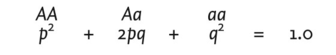# Hardy-Weinberg law

Also found in: Dictionary, Encyclopedia, Wikipedia.

## Har·dy-Wein·berg law

(har'dē wīn'bĕrg),
if mating occurs at random with respect to any one autosomal locus in a population in which the gene frequencies are equal in the two sexes, and the factors tending to change gene frequencies (mutation, differential selection, migration) are either absent or negligible, then in one generation the probabilities of all possible genotypes will on average equal the same proportions as if the genes were assembled at random. The law does not apply to two or more loci jointly, nor to X-linked traits where the initial gene frequencies differ in the two sexes.
Farlex Partner Medical Dictionary © Farlex 2012

## Hardy-Weinberg law

(här′dē-wīn′bûrg)
n.
A fundamental principle in population genetics stating that the genotype frequencies and gene frequencies of a large, randomly mating population remain constant provided immigration, mutation, and selection do not take place.

## Har·dy-Wein·berg law

(hahr'dē wīn'bĕrg law)
If mating occurs at random with respect to any one autosomal locus in a population in which the gene frequencies are equal in the two sexes, and the factors tending to change gene frequencies (mutation, differential selection, migration) are either absent or negligible, then in one generation the probabilities of all possible genotypes will on average equal the same proportions as if the genes were assembled at random. The law does not apply to two or more loci jointly, nor to X-linked traits where the initial gene frequencies differ in the two sexes.
Medical Dictionary for the Health Professions and Nursing © Farlex 2012

## Hardy-Weinberg law

a law stating that in a randomly mating large population the frequency of ALLELES will remain constant from generation to generation. Proposed in 1908 by G.H. Hardy in England and W. Weinberg in Germany, the law explains how the various alleles of a gene could remain constant in a population and yet be inherited by the rules of MENDELIAN GENETICS. The law shows why, for instance, dominant PHENOTYPES are not necessarily in excess of recessive ones in a population. If a gene has two alleles, A and a , with a frequency of p and q respectively, the genotypic frequencies would have the proportions:This equation is derived from the BINOMIAL EXPANSION (p + q)2 = 1.0. Such GENETIC EQUILIBRIUM only occurs under the following conditions: zero selection and mutation, no emigration or immigration, random mating and a large population size. However, selection pressures can produce stability (see GENETIC POLYMORPHISM and selection and mutation effects might cancel each other out.

Collins Dictionary of Biology, 3rd ed. © W. G. Hale, V. A. Saunders, J. P. Margham 2005

## Hardy,

Godfrey H., English mathematician, 1877-1947.
Hardy-Weinberg equilibrium - the state in which the genetic structure of the population conforms to the prediction of the Hardy-Weinberg law. Synonym(s): random mating equilibrium
Hardy-Weinberg law - if mating occurs at random with respect to any one autosomal locus in a population in which the gene frequencies are equal in the two sexes, and the factors tending to change gene frequencies are absent or negligible, then in one generation the probabilities of all possible genotypes will on average equal the same proportions as if the genes were assembled at random.

## Weinberg,

Wilhelm, German physician, 1862-1937.
Hardy-Weinberg equilibrium - see under Hardy, Godfrey H
Hardy-Weinberg law - see under Hardy, Godfrey H